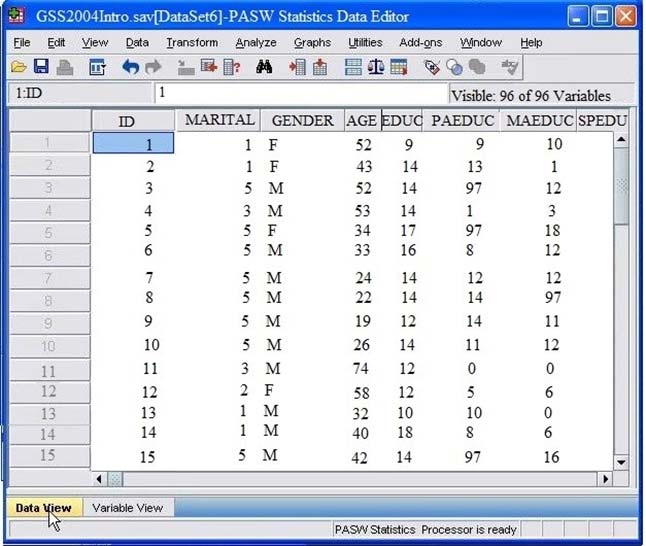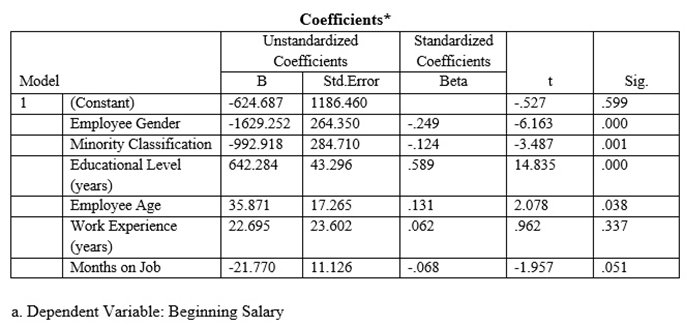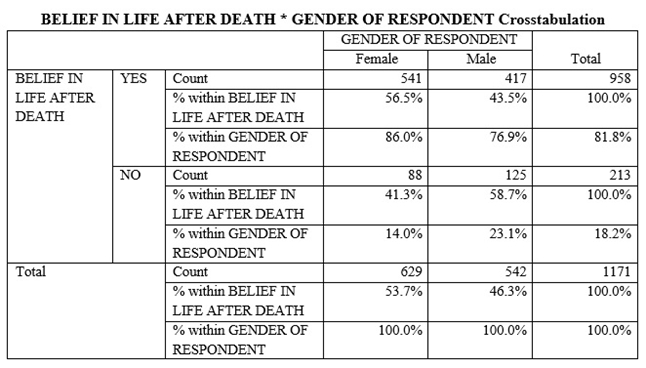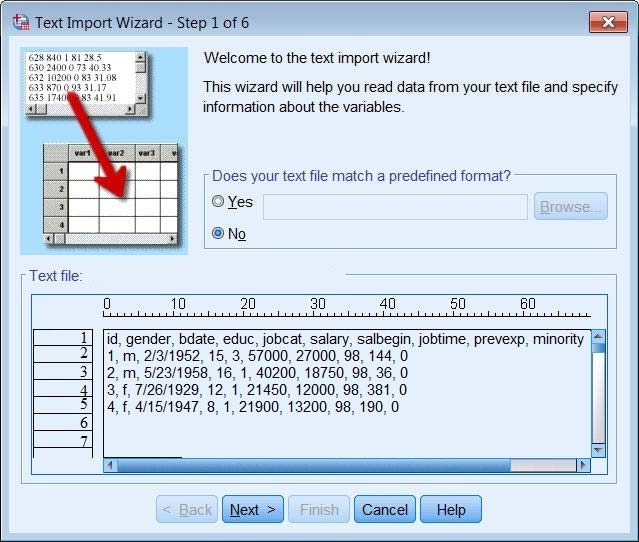# IBM SPSS Statistics Level 1 v2 v1.0

Page:    1 / 4
Exam contains 58 questions

Which transformation feature would you use to convert a string variable with values such as Female and Male to a numeric variable with values 1 and 2?

• A. Define Variable Properties
• B. Automatic Recode
• C. Visual Binning
• D. Shift Values

Consider the variable AGE in the data file below. You have been asked to use this in a cross-tabulation with GENDER. How would you proceed? (Choose two.)• A. Go to Analyze"¦ Descriptive Statistics"¦ Crosstabs"¦, specify the variables and click OK.
• B. Use Visual Binning to create a categorical equivalent for AGE and proceed as per Option A. above.
• C. Use Visual Binning to create a categorical equivalent for AGE and run a Frequencies.
• D. Use Recode into Different Variables"¦ to create a categorical equivalent for AGE and proceed as per Option A. above.

Which interpretation is correct for this Regression Coefficients table?• A. For every additional year that you get older, your beginning salary decreases on average by \$.13.
• B. For every additional year that you get older, your beginning salary decreases on average by \$35.9.
• C. For every additional year that you get older, your beginning salary increases on average by \$.13.
• D. For every additional year that you get older, your beginning salary increases on average by \$35.9.

What happens when you use the Output option Filter out unselected cases in the Select Cases"¦ dialog to create a filter variable and retain all cases in the same data file? is created. Cases with a value of 1 for this variable have a slash through their row number, indicating they are not selected. is created. Cases with a value of 0 for this variable have a slash through their row number, indicating they are not unselected and appears on the status bar of the Data Editor. is created. Cases with a value of 0 for this variable have a slash through their row number, indicating they are unselected and the appears on the status bar of the Data Editor. is created. Cases with a value of 1 for this variable have a slash through their row number, indicating they are unselected and the appears on the status bar of the Data Editor.

With IBM SPSS Statistics you can assign one of three levels of measurement to a variable. Which is the correct list of all three levels?

• A. Nominal-Ordinal-Interval
• B. Categorical-Interval-Ratio
• C. Nominal-Ordinal-Scale
• D. Nominal-Interval-Scale

The Date and Time Wizard can be used to do which operation? (Choose all that apply.)

• A. Create a date/time variable from a string variable containing a date and time.
• B. Construct a date/time variable by merging variables containing different parts of the date or time.
• C. Add or subtract values from date/time variables, including add or subtracting two date/time variables.
• D. Extract a part of a date or time variable.

Which feature would you use to create a new variable whose values represent the number of times that each respondent answered Strongly Agree to a series of satisfaction questions?

• A. Compute variable
• B. Visual Binning
• C. Count Values within Cases
• D. Rank Cases

Which operations are available from the File menu?

• A. Open and Save data files
• B. Print the contents of the active IBM SPSS Statistics window
• C. Exit IBM SPSS Statistics
• D. Run the FREQUENCIES procedure

Which statement is the correct interpretation of this crosstab table?• A. 56.5% of females believe in life after death
• B. 86.0% of females believe in life after death
• C. 27.5% of females believe in life after death
• D. 53.7% of females believe in life after death

The Merge Files facility is located under which menu?

• A. View
• B. Transform
• C. Analyze
• D. Data

True or false: the text file shown in the figure below is an example of a fixed format text file.• A. True
• B. False

What information is contained in the journal file?

• A. Information relating to the IBM SPSS Statistics data file you are working with
• B. Information relating to the IBM SPSS Statistics data file you are working with and any output created
• C. Syntax from all operations relating to manipulating variables
• D. Syntax from actions taken in dialog boxes or from running commands from the Syntax Editor

Which variable name is correctly formed for use in an IBM SPSS Statistics data file?

Which statement is true about IQR (interquartile range)? (Choose two.)

• A. It is used to measure the spread of the data
• B. It is equal to the mode th th
• C. It is the range between the values at the 25 and 75 percentile
• D. It indicates whether the variable is normally distributed or not

The IBM SPSS Statistics Select Cases provides various ways to select cases included in further analysis. If you needed to conduct analysis on those respondents whose age is more than 30 years, earning in excess of \$40000, which option in this dialog would you choose to specify these criteria?

• A. Random sample of cases
• B. Based on time or case range
• C. Use filter variable
• D. If condition is satisfied